## Classification

# of variables # of modes # of jumps
2 1 1
Type Continuous dynamics Guards & Invariants Resets
hybrid linear polynomial linear polynomial linear polynomial

 Flow* model I neuron_I.model Flow* model II neuron_II.model

## Description of model I

The general dynamics of the model of spiking neurons is defined by the following ODE.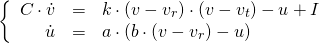wherein the constant parameters are given by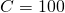,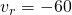,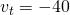,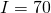,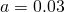and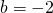. The value of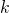is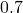when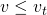, otherwise it is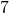. Whenever the value of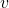reaches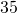, its value is reset to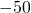and meanwhile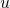is updated to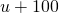.

## Reachability settings for model I

We consider the initial set defined by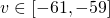,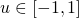.

## Results for model I

The following figures show an overapproximation computed by Flow* for the time horizon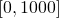.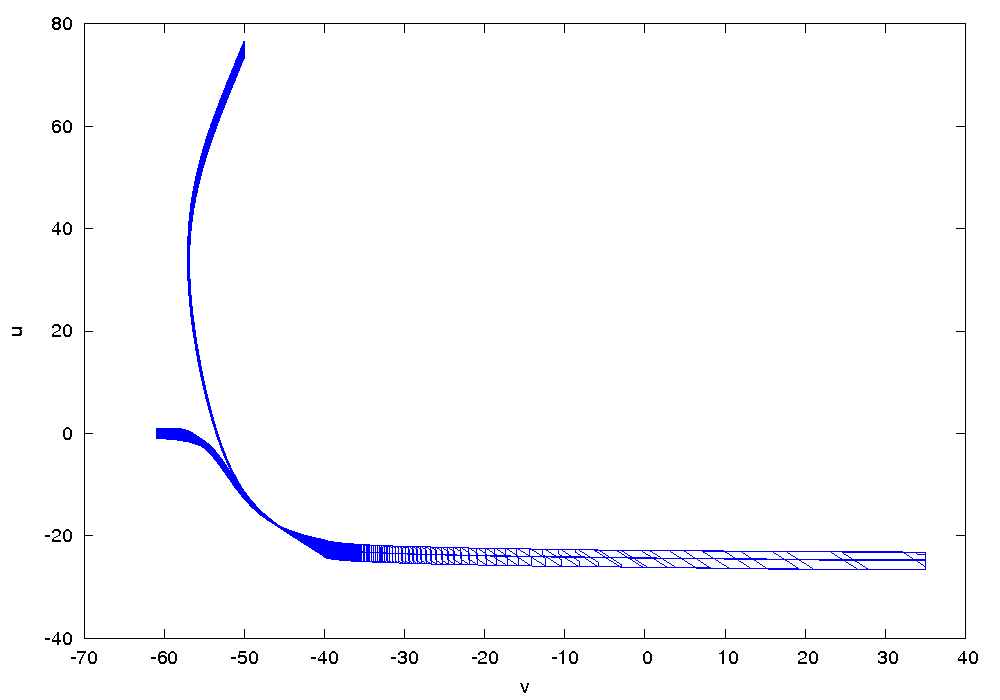## Description of model II

As the second example, the constant parameters are given by,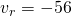,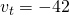,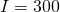,,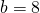and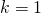. The values of,are reset to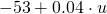and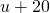respectively when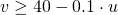.

## Reachability settings for model II

We consider the initial set defined by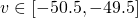,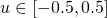.

## Results for model II

The following figures show an overapproximation computed by Flow* for the time horizon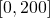.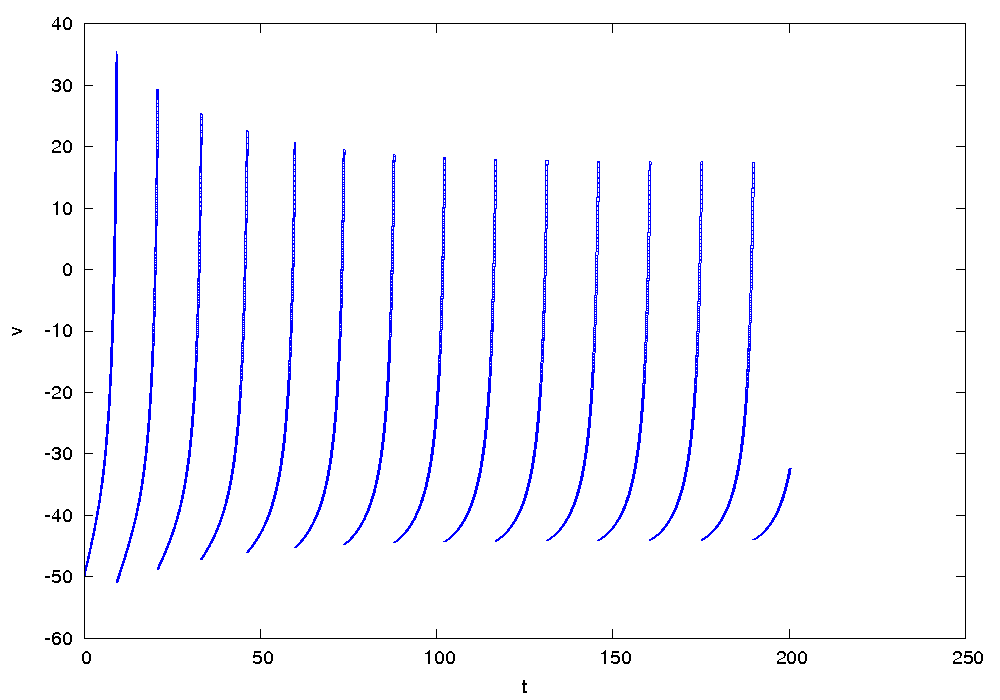## References

 E. Izhikevich. Dynamical Systems in Neuroscience: The Geometry of Excitability and Bursting. The MIT Press, 2010.